# Circle Problems

#### Number of problems found: 502

• Rewrite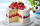Rewrite the following as division statement  if there are 8 cakes and each person get 1/12 of a cake how many people get cake?
• A circle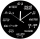A circle relation is given to be x2 + y2 =16. What is the radius of the circle?
• The spinner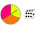The spinner below is spun 12 times. It landed on I 4 times, II 7 times, and III 1 time. What is the difference between the experimental and theoretical probabilities of landing on the II?
• Wheel gearA drive wheel of radius 2 is connected to a drive wheel of radius 1 by a pulley of length 17. What is the distance between the wheel axles?
• One ballOne ball of dough can be stretched into a circle to make a pizza. After the pizza is cooked, it is cut into 8 equal slices. How many slices of pizza can you make with 3 balls of dough? Solve this problem any way you choose.
• Round cakeWhat is the area of a round cake with a radius of 5cm?
• Pumpkin pie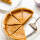Have some pumpkin pie. One half of the pie is cut into 4 equal slices, the other half is cut into 3 equal slices. After eating one of the larger slices (on the 3 piece side), I wonder if I will eat more if I have one more from the 3 side, or two from the
• Length of the chord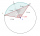Calculate the length of the chord in a circle with a radius of 25 cm with a central angle of 26°.
• Minute handWhat is the distance the minute hand of the clock travels in 12 minutes, if the diameter of the clock is 30 cm and the hand extends to a distance of 2 cm from the edge of the clock?
• The volleyball ball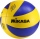The volleyball ball can have a circumference after inflation of at least 650 max 750 mm. What volume of air can this ball hold, if its circumference is the average of the minimum and maximum inflation of the ball.
• Surveyors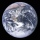Surveyors mark 4 points on the surface of the globe so that their distances are the same. What is their distance from each other?
• The collar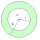The collar on the dress has the shape of an annulus 6 cm wide. The circumference of the inner circle is 31.4 cm. How much cm2 of fabric is needed to make one collar?
• Top-open tank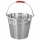The top-open tank has the shape of a truncated rotating cone, which stands on a smaller base. The tank's volume is 465 m3, the radii of the bases are 4 m and 3 m. Find the depth of the tank.
• Metal ballsFour metal balls with a diameter of 5 cm are placed in a measuring cylinder with an inner diameter of 10 cm. What is the smallest water volume to be poured into the cylinder so that all balls are below the water level?
• Woman's day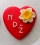We can easily make a heart for mothers for Woman's day by drawing two semicircles to the two upper sides of the square standing on their top. What is the radius of the circle circumscribed by this heart when the length of the side of the square is 1?
• Tarzan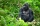Tarzan, weighing m = 90 kg, tries to cross the river by swinging with a vine hanging from a tree leaning over the river. Liana has a length l = 12 m. The speed of the Tarzan in the lowest swing position is v = 8 m/s. Tarzan doesn't know that the liana wil
• MegapizzaMegapizza will be divided among 100 people. First gets 1%, 2nd 2% of the remainder, 3rd 3% of the remainder, etc. Last 100th 100% of the remainder. Which person got the biggest portion?
• I need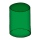I need to calculate the height of the cylinder. I have a given that the radius is 6 cm and the volume is 282.6 cm3. What is the formula for this?
• Largest possible cone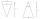It is necessary to make the largest possible cone from an iron rod in the shape of a prism with dimensions of 5.6 cm, 4.8 cm, 7.2 cm. a) Calculate its volume. b) Calculate the waste.
• Metal washersMetal washers with a diameter of 80 mm are cut from a strip of steel sheet with a width of 10 cm and a length of 2 m. Calculate the percentage of material waste if no material is lost when two adjacent circles meet.

Do you have an exciting math question or word problem that you can't solve? Ask a question or post a math problem, and we can try to solve it.

We will send a solution to your e-mail address. Solved examples are also published here. Please enter the e-mail correctly and check whether you don't have a full mailbox.

Please do not submit problems from current active competitions such as Mathematical Olympiad, correspondence seminars etc...